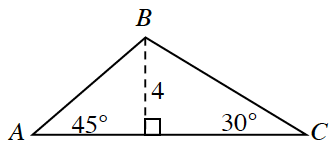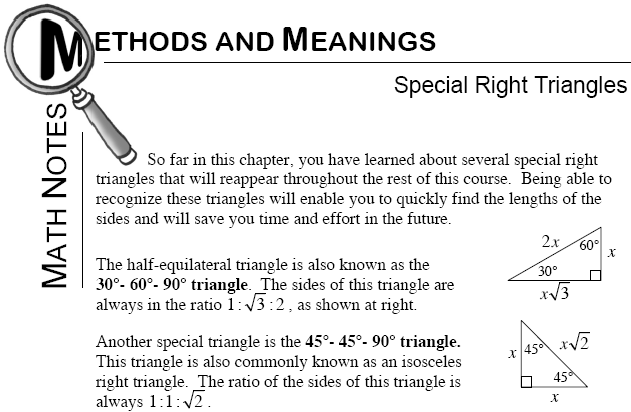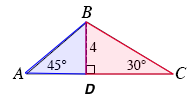### Home > GC > Chapter 5 > Lesson 5.3.5 > Problem5-122

5-122.

Find the area and perimeter of $ΔABC$ below. Give approximate (decimal) answers, not exact answers. Homework Help ✎Separate $ΔABC$ into two separate triangles by dropping the height from vertex $B$.
Label the new point $D$, creating $ΔABD$ and $ΔDBC$.

Both of the triangles that have been created are special right triangles, because the height $\overline{BD}$ creates two right triangles: a $45º-45º-90º$ triangle and a $30º-60º-90º$ triangle.

Refer to the Math Notes box below to find the lengths of the unknown sides.
Then use this information to find the area and perimeter of the original triangle.